# Use implicit differentiation to find the equation of the tangent line to the curve xy^3+2xy=9 at the point (31)

Use implicit differentiation to find the equation of the tangent line to the curve xy^3+2xy=9 at the point (31). The equation of this tangent line can be written in the form y=mx+b where m is

x(3y^2dy) + y^3(dx) +2xdy + 2ydx = 0

dy (3xy^2+2x) = -dx (y^3+2y)

dy/dx = -(y^3+2y)/(3xy^2+2x)

I assume you mean the point (3,1)
x = 3
y = 1
so
m = dy/dx = -(1+2)/(9+6) = - 3/15 = -1/5
put in point
1 = m * 3 + b
1 = -3/5 + b
b = 8/5
y = -x/5 + 8/5
5y = 8-x

#### Earn Coin

Coins can be redeemed for fabulous gifts.

Similar Homework Help Questions
• ### Use implicit differentiation to find the equation of the tangent line to the curve xy^3+xy=5 at the point (4,1)

Use implicit differentiation to find the equation of the tangent line to the curve xy^3+xy=5 at the point (4,1) . The equation of this tangent line can be written in the form y = mx+b where m is:? and where b is:?

• ### Use implicit differentiation to find the equation of the tangent line to the curve xy3+xy=14 at the point (7,1)

Use implicit differentiation to find the equation of the tangent line to the curve xy3+xy=14 at the point (7,1) . The equation of this tangent line can be written in the form y=mx+bi dont seem to no how to find m or b

• ### (1 point) Use implicit differentiation to find an equation of the tangent line to the curve...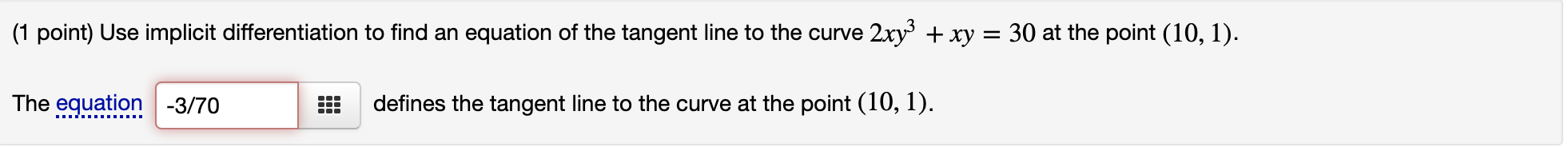(1 point) Use implicit differentiation to find an equation of the tangent line to the curve 2xy3+xy=302xy3+xy=30 at the point (10,1)(10,1). (1 point) Use implicit differentiation to find an equation of the tangent line to the curve 2xy3 + xy = 30 at the point (10, 1). The equation -3/70 defines the tangent line to the curve at the point (10, 1).

• ### Find the equation of the tangent line to the curve at the given point using implicit...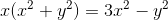Find the equation of the tangent line to the curve at the given point using implicit differentiation. Remember: equation of a line can be found by y-y1=m(x-x1) where m is the slope of the line and (x1,y1) is any point on the line. Curve: at (1,1)

• ### Problem 3. (1 point) Find the equation of the line that is tangent to the curve y = 4x cos x at t...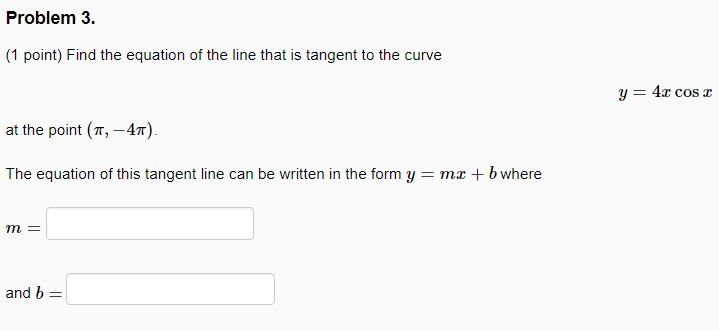Problem 3. (1 point) Find the equation of the line that is tangent to the curve y = 4x cos x at the point (π,-47) The equation of this tangent line can be written in the form y mx b where and b Problem 3. (1 point) Find the equation of the line that is tangent to the curve y = 4x cos x at the point (π,-47) The equation of this tangent line can be written in the form...

• ### Find the equation of the tangent line to the curve y=6tanx at the point (pi/4,6)

Find the equation of the tangent line to the curve y=6tanx at the point (pi/4,6). The equation of this tangent line can be written in the form y=mx+b where m is:and where b is:

• ### Find the equation of the tangent line to the curve y=5xcosx at the point (pi,–5pi)

Find the equation of the tangent line to the curve y=5xcosx at the point (pi,–5pi).The equation of this tangent line can be written in the form y=mx+b wherem=and b=

• ### Find the equation of the tangent line to the curve y = 6 sin z at...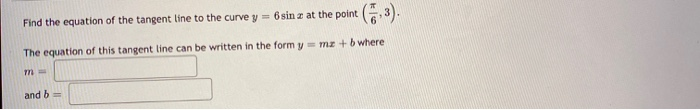Find the equation of the tangent line to the curve y = 6 sin z at the point (6-3). The equation of this tangent line can be written in the form y = mx + b where m- and b =

• ### Use implicit differentiation to find the slope of the tangent line to the curve 5x^3 y^2...

Use implicit differentiation to find the slope of the tangent line to the curve 5x^3 y^2 - 4x^2 y = 1at the point (1,1) m=

• ### 3. Use implicit differentiation for the curve x3 + 3y4 = xy and then find the...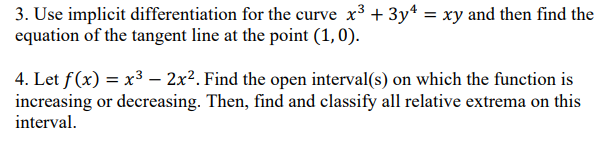3. Use implicit differentiation for the curve x3 + 3y4 = xy and then find the equation of the tangent line at the point (1,0). 4. Let f(x) = x3 – 2x2. Find the open interval(s) on which the function is increasing or decreasing. Then, find and classify all relative extrema on this interval